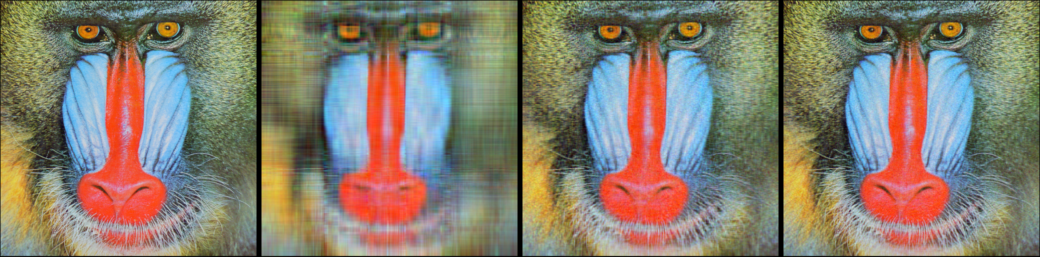This demonstration shows how to work with color channels to explore image compression using the Singular Value Decomposition (SVD).

In :
using Images, TestImages
using LinearAlgebra

img = float.(testimage("mandrill"))
channels = channelview(img)

function rank_approx(F::SVD, k)
U, S, V = F
M = U[:, 1:k] * Diagonal(S[1:k]) * V[:, 1:k]'
clamp01!(M)
end

Out:
rank_approx (generic function with 1 method)

For each channel, we do SVD decomposition, and then reconstruct the channel using only the K largest singular values.

The image is compressed because for each channel we only need to save two small matrices and one vector -- truncated part of (U, S, V). For example, if the original image is gray image of size (512, 512), and we rebuild the image with $50$ singular values, then we only need to save $2 \times 512 \times 50 + 50$ numbers to rebuild the image, while original image has $512 \times 512$ numbers. Hence this gives us a compression ratio $19.55\%$ if we don't consider the storage type.

In :
# before julia v1.1:
# svdfactors = (svd(channels[1,:,:]), svd(channels[2,:,:]), svd(channels[3,:,:]))
svdfactors = svd.(eachslice(channels; dims=1))
imgs = map((10, 50, 100)) do k
colorview(RGB, rank_approx.(svdfactors, k)...)
endFrom left to right: original image, reconstructed images using 10, 50, 100 largest singular values. We can see that $50$ largest singular values are capable of rebuilding a pretty good image.